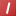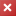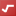# Mathematical Functoids Reference

Use Mathematical functoids to perform a variety of basic mathematical operations.

Important

Because Microsoft BizTalk Server uses the underlying functionality of the .NET Framework, the results produced by some of the Mathematical functoids may vary from the equivalent functoids in earlier versions of BizTalk Server. You should test your maps thoroughly to ensure that you are getting the results you expect.

When a Mathematical functoid determines that one or more of its input parameters is not valid, it cancels the operation and returns an empty string, such that a field in an output instance message is created as "<FieldName></FieldName>" and the string Size functoid returns zero (0).

When the input parameter descriptions specify a numeric value, strings that can be interpreted as numbers are also accepted.

For conceptual information about Mathematical functoids, see Mathematical Functoids.

The following table shows the functoids in the Mathematical category.

Mathematical functoid DescriptionAbsolute Value Calculates the absolute value of the specified value.Addition Calculates the sum of the numeric input parameters.Division Calculates the quotient of the specified dividend and divisor.Integer Returns the integer portion of a numeric input parameter.Maximum Value Determines the largest value among the numeric input parameters.Minimum Value Determines the smallest value among the numeric input parameters.Modulo Calculates the remainder of the specified integer division.Multiplication Calculates the product of the numeric input parameters.Round Rounds the specified numeric input parameter to the nearest specified number of decimal places.Square Root Calculates the square root of a specified numeric value.Subtraction Calculates the result of subtracting up to 99 numeric values from another numeric value.